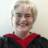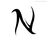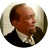Trying to write basic mathematical formula for InventoryI need a sum so that the display shows the Remaining Stock

Fields are currently numeric for "Number" and "Supplied"

eg:  Number = 50, Supplied = 3, so Remaining Stock = 47

I had:  Remaining Stock = Number - Supplied

HELP!Hi,

the normal way to count Inventory Items is:

Remaining Stock = UnitsInStock + sum(PurchaseInvoice.Supplied) - sum(SalesInvoice.Sold)

NickThat's too complicated.  I ended up just using a field with Number-Supplied!it has to be:

`–––`
`Remaining Stock := Number - Supplied`
`–––`

Birger